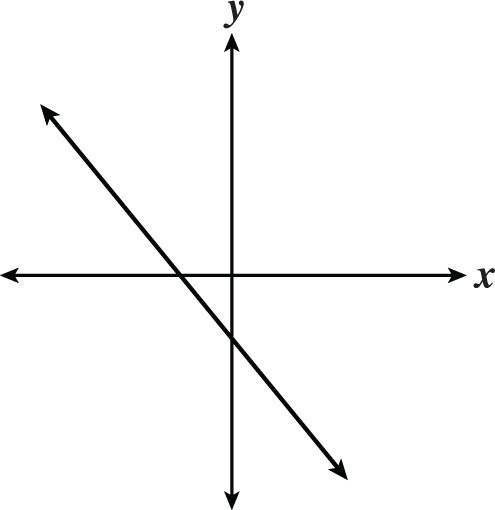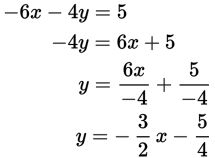# SAT Math Multiple Choice Question 310: Answer and Explanation

### Test Information

Question: 310

10.The graph above could represent which of the following equations?

• A. -6x - 4y = 5
• B. -6x - 4y = -5
• C. -6x + 4y = 5
• D. -6x + 4y = -5

Explanation:

A

Difficulty: Medium

Category: Heart of Algebra / Linear Equations

Strategic Advice: Notice that there are no grid-lines and no numbers on the axes. This is a great clue that the numbers in the equations don't actually matter.

Getting to the Answer: The line is decreasing, so the slope (m) is negative. The line crosses the y-axis below 0, so the y-intercept (b) is also negative. Put each answer choice in slope-intercept form, one at a time, and examine the signs of m and b. Begin with A:You don't need to check any of the other equations. Choice (A) has a negative slope and a negative y-intercept, so it is the correct equation.